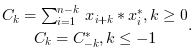Scilab Home page | Wiki | Bug tracker | Forge | Mailing list archives | ATOMS | File exchange
Change language to: English - Português - 日本語 - Русский

xcorr

Computes discrete auto or cross correlation

Syntax

[c, lagindex] = xcorr(x)
[c, lagindex] = xcorr(x, y)
[c, lagindex] = xcorr(.., maxlags)
[c, lagindex] = xcorr(.., maxlags, scaling)

Parameters

x

a vector of real or complex floating point numbers.

y

a vector of real or complex floating point numbers. The default value is x.

maxlags

a scalar with integer value greater than 1. The default value is n. Where n is the maximum of the x and y vector length.

scaling

a character string with possible value: "biased", "unbiased", "coeff", "none". The default value is "none".

c

a vector of real or complex floating point numbers with same orientation as x.

lagindex

a row vector, containing the lags index corresponding to the c values.

Description

• c=xcorr(x) computes the un-normalized discrete auto correlation:and return in c the sequence of auto correlation lags Ck=-n:n where n is the length of x
• xcorr(x,y) computes the un-normalized discrete cross correlation:and return in c the sequence of auto correlation lags Ck=-n:n where n is the maximum of x and y lengths.

If the maxlags argument is given xcorr returns in c the sequence of auto correlation lags Ck=-maxlags:maxlags. If maxlags is greater than length(x), the first and last values of c are zero.

The scaling argument describes how C(k) is normalized before being returned in c:

• "biased": c=C/n.
• "unbiased": c=C./(n-(-maxlags:maxlags)).
• "coeff": c=C/(norm(x)*norm(y)).The corr function computes the "biased" covariance of x and y and only return in c the sequence of auto correlation lags Ck≥0. Method: This function computes C using ifft(fft(x).*conj(fft(y))).

Examples

t = linspace(0, 100, 2000);
y = 0.8 * sin(t) + 0.8 * sin(2 * t);
[c, ind] = xcorr(y, "biased");
plot(ind, c)• xcov — Computes discrete auto or cross covariance
• corr — correlation, covariance
• fft — Transformée de Fourier discrète rapide.